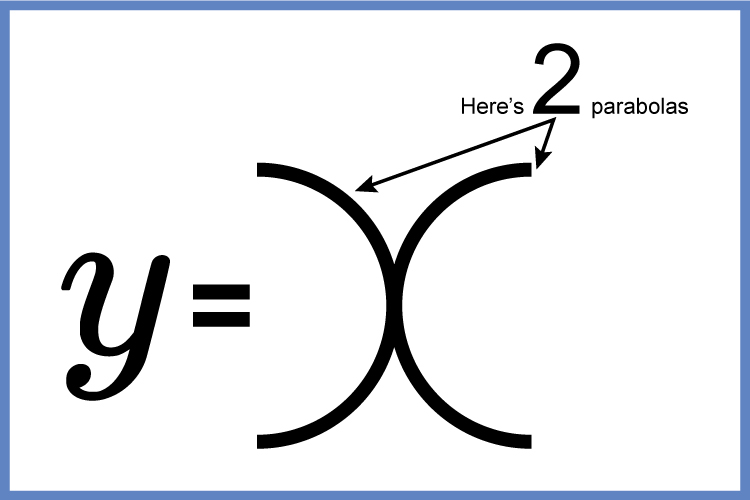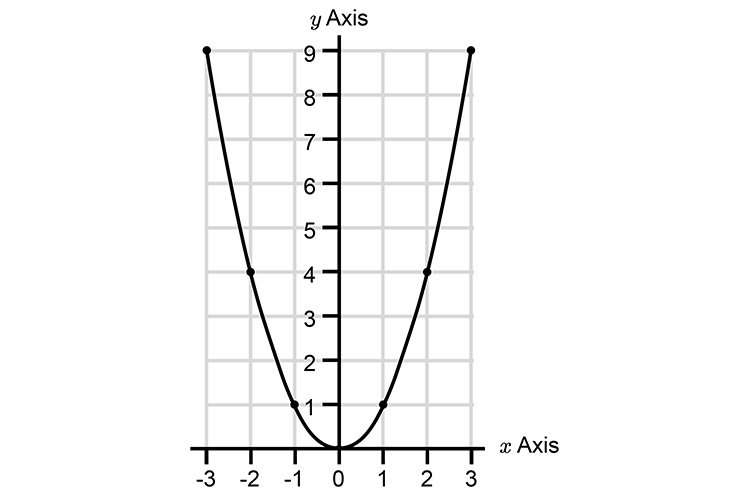# Manipulating parabolas

## The most famous parabola

In any parabola question always remember the most famous parabola, this will help you learn what happens with transforming parabolas.

y=x^2

To remember the most famous parabola:Why! Even x  has 2  parabolas (y=x^2)

Now plot the parabola y=x^2

Try values of x

 x=3 Then y= 3^2 =9 x=2 Then y= 2^2 =4 x=1 Then y= 1^2 =1 x=0 Then y= 0^2 =0 x=-1 Then y= -1^2 =1 x=-2 Then y= -2^2 =4 x=-3 Then y= -3^2 =9

And if we plot thisHere is the most famous parabola in the world plotted out.